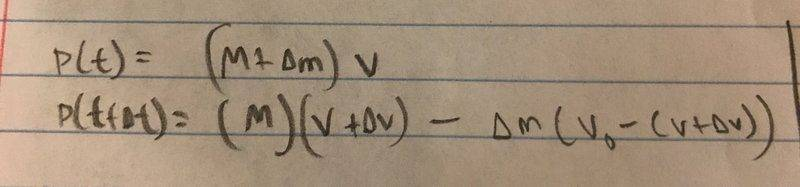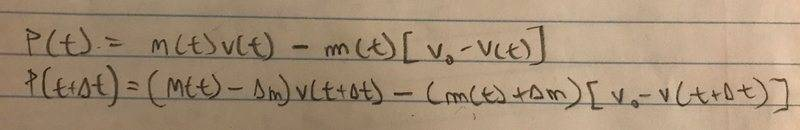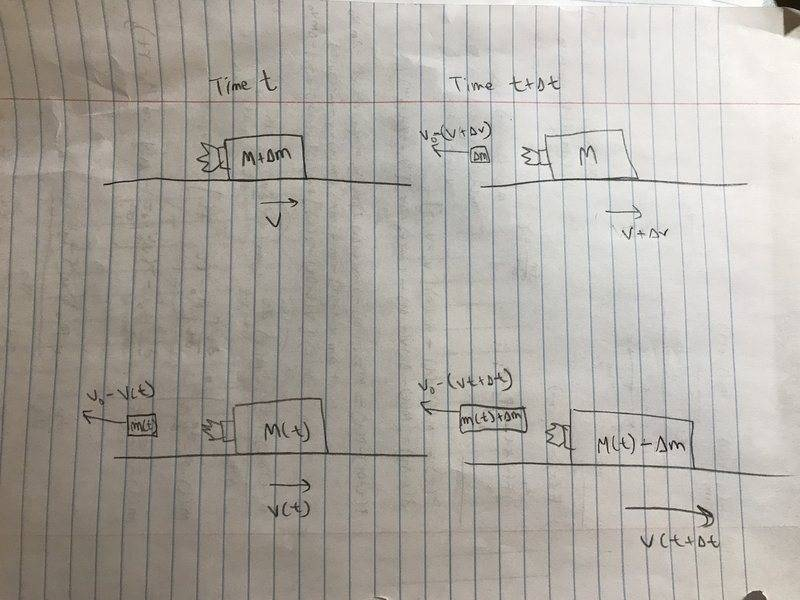# Momentum Differential Equation

## Homework Statement

A rocket sled moves along a horizontal plane, and is retarded by a friction force friction = μW, where μ is constant and W is the weight of the sled. The sled’s initial mass is M, and its rocket engine expels mass at constant rate dM/dt ≡ γ; the expelled mass has constant speed v0 relative to the rocket. The rocket sled starts from rest and the engine stops when half the sled’s total mass is gone. Find an expression for the maximum speed.

d(mv)/dt = F(x)

## The Attempt at a Solution

To solve the problem, I found the differential equation for momentum, taking the momentum at a point in time and then the momentum a moment later, then dividing by a change in time.

This interpretation worked.This interpretation did not work.I don't understand why the second interpretation does not work.

Thanks

haruspex
Homework Helper
Gold Member
2020 Award

## Homework Statement

A rocket sled moves along a horizontal plane, and is retarded by a friction force friction = μW, where μ is constant and W is the weight of the sled. The sled’s initial mass is M, and its rocket engine expels mass at constant rate dM/dt ≡ γ; the expelled mass has constant speed v0 relative to the rocket. The rocket sled starts from rest and the engine stops when half the sled’s total mass is gone. Find an expression for the maximum speed.

d(mv)/dt = F(x)

## The Attempt at a Solution

To solve the problem, I found the differential equation for momentum, taking the momentum at a point in time and then the momentum a moment later, then dividing by a change in time.

This interpretation worked.

View attachment 211726

This interpretation did not work.

View attachment 211727

I don't understand why the second interpretation does not work.

Thanks
I do not understand how you obtained the first equation of the second method. What is your reasoning?

I do not understand how you obtained the first equation of the second method. What is your reasoning?

I set up a differential equation for the change in momentum in a small change in time, according to the diagrams below. The second setup, when I solve, gives the wrong answer.haruspex
Homework Helper
Gold Member
2020 Award
I set up a differential equation for the change in momentum in a small change in time, according to the diagrams below. The second setup, when I solve, gives the wrong answer.

View attachment 211728
In the lower left diagram, you have a mass m(t). This appears to be the total mass of fuel burnt so far. But you do not know its total momentum. It is not all at velocity v0-v(t) since v(t) has been changing.

•ecoo
In the lower left diagram, you have a mass m(t). This appears to be the total mass of fuel burnt so far. But you do not know its total momentum. It is not all at velocity v0-v(t) since v(t) has been changing.

I see what you mean, but isn't that taken care of when we have change in time approach 0 (this is my next step to find an expression for change in momentum). And why would that not also apply to the first scenario, where fuel is also leaving while the rocket accelerates?

Last edited:
haruspex
Homework Helper
Gold Member
2020 Award
isn't that taken care of when we have change in time approach 0
Perhaps, except that if you expand the second equation you find a term m(t)v(t+Δt). That is multiplying the fuel already burnt by the increased velocity, which doesn't make much sense.

•ecoo
Perhaps, except that if you expand the second equation you find a term m(t)v(t+Δt). That is multiplying the fuel already burnt by the increased velocity, which doesn't make much sense.

Thank you for the help. I now understand what you were saying before.